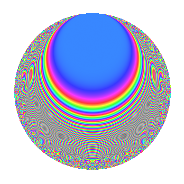# Properties

 Label 287.2.h.dLevel 287 Weight 2 Character orbit 287.h Analytic conductor 2.292 Analytic rank 0 Dimension 40 CM No

# Related objects

## Newspace parameters

 Level: $$N$$ = $$287 = 7 \cdot 41$$ Weight: $$k$$ = $$2$$ Character orbit: $$[\chi]$$ = 287.h (of order $$5$$ and degree $$4$$)

## Newform invariants

 Self dual: No Analytic conductor: $$2.29170653801$$ Analytic rank: $$0$$ Dimension: $$40$$ Relative dimension: $$10$$ over $$\Q(\zeta_{5})$$ Sato-Tate group: $\mathrm{SU}(2)[C_{5}]$

## $q$-expansion

The dimension is sufficiently large that we do not compute an algebraic $$q$$-expansion, but we have computed the trace expansion.

 $$\operatorname{Tr}(f)(q) =$$ $$40q - 2q^{2} - 10q^{3} - 14q^{4} - q^{5} + 9q^{6} + 10q^{7} + 3q^{8} + 22q^{9} + O(q^{10})$$ $$\operatorname{Tr}(f)(q) =$$ $$40q - 2q^{2} - 10q^{3} - 14q^{4} - q^{5} + 9q^{6} + 10q^{7} + 3q^{8} + 22q^{9} + 10q^{10} + 5q^{11} - 17q^{12} + 3q^{13} - 8q^{14} + 23q^{15} - 18q^{16} + 11q^{17} - 38q^{18} - 2q^{19} + 31q^{20} + 4q^{22} + 2q^{23} + 10q^{24} - 21q^{25} - 7q^{26} - 52q^{27} + 14q^{28} - 11q^{29} - 18q^{30} - 3q^{31} + 44q^{32} - 51q^{33} + 29q^{34} - 9q^{35} + 35q^{36} + 11q^{37} + 52q^{38} - 5q^{39} - 32q^{40} + 29q^{41} + 6q^{42} - 32q^{43} - 92q^{44} - 56q^{45} + 26q^{46} + 29q^{47} + 11q^{48} - 10q^{49} - 24q^{50} - 4q^{51} + 3q^{52} + 30q^{53} + 58q^{54} - 100q^{55} - 3q^{56} - 49q^{57} + 25q^{58} + 5q^{59} - 91q^{60} + 22q^{61} - 34q^{62} + 13q^{63} - 9q^{64} + 21q^{65} + 29q^{66} + 9q^{67} - 20q^{68} + 30q^{69} - 10q^{70} + 34q^{71} - 37q^{72} - 20q^{73} - 58q^{74} + 41q^{75} - 37q^{76} - 5q^{77} + 63q^{78} + 66q^{79} + 22q^{80} + 96q^{81} + 76q^{82} - 22q^{83} - 38q^{84} - 26q^{85} + 3q^{86} + 49q^{87} - 19q^{89} - q^{90} + 22q^{91} - 2q^{92} - 39q^{93} + 66q^{94} + 71q^{95} - 302q^{96} + 47q^{97} - 2q^{98} + 28q^{99} + O(q^{100})$$

## Embeddings

For each embedding $$\iota_m$$ of the coefficient field, the values $$\iota_m(a_n)$$ are shown below.

For more information on an embedded modular form you can click on its label.

Label $$a_{2}$$ $$a_{3}$$ $$a_{4}$$ $$a_{5}$$ $$a_{6}$$ $$a_{7}$$ $$a_{8}$$ $$a_{9}$$ $$a_{10}$$
57.1 −0.837127 + 2.57641i 0.305172 −4.31909 3.13800i −1.36956 0.995044i −0.255467 + 0.786248i −0.309017 0.951057i 7.31716 5.31623i −2.90687 3.71014 2.69558i
57.2 −0.514559 + 1.58365i 1.87936 −0.625144 0.454194i 2.01594 + 1.46467i −0.967042 + 2.97625i −0.309017 0.951057i −1.65331 + 1.20120i 0.531995 −3.35684 + 2.43889i
57.3 −0.509577 + 1.56832i −1.29067 −0.581915 0.422786i 0.393282 + 0.285736i 0.657694 2.02417i −0.309017 0.951057i −1.70859 + 1.24136i −1.33418 −0.648532 + 0.471186i
57.4 −0.454415 + 1.39854i −3.02432 −0.131400 0.0954680i −2.14566 1.55892i 1.37430 4.22965i −0.309017 0.951057i −2.18612 + 1.58831i 6.14653 3.15524 2.29241i
57.5 0.0642451 0.197726i −0.765544 1.58307 + 1.15016i 3.13381 + 2.27685i −0.0491825 + 0.151368i −0.309017 0.951057i 0.665514 0.483524i −2.41394 0.651524 0.473360i
57.6 0.286127 0.880607i −2.35844 0.924433 + 0.671640i −0.331415 0.240787i −0.674812 + 2.07686i −0.309017 0.951057i 2.35413 1.71038i 2.56223 −0.306866 + 0.222951i
57.7 0.491871 1.51382i 0.558674 −0.431686 0.313638i −3.26045 2.36885i 0.274795 0.845733i −0.309017 0.951057i 1.88834 1.37196i −2.68788 −5.18974 + 3.77057i
57.8 0.567566 1.74679i 2.49604 −1.11111 0.807268i 0.226121 + 0.164287i 1.41667 4.36005i −0.309017 0.951057i 0.931061 0.676455i 3.23020 0.415314 0.301743i
57.9 0.718417 2.21106i 0.707185 −2.75463 2.00136i 3.19921 + 2.32437i 0.508054 1.56363i −0.309017 0.951057i −2.64242 + 1.91983i −2.49989 7.43769 5.40380i
57.10 0.805486 2.47903i −2.12549 −3.87876 2.81808i −0.434226 0.315483i −1.71205 + 5.26916i −0.309017 0.951057i −5.89282 + 4.28139i 1.51771 −1.13186 + 0.822342i
78.1 −2.21373 + 1.60837i −3.42753 1.69571 5.21887i −0.479452 + 1.47560i 7.58764 5.51274i 0.809017 + 0.587785i 2.94888 + 9.07572i 8.74800 −1.31193 4.03772i
78.2 −2.02082 + 1.46821i 0.931822 1.31004 4.03189i 0.525509 1.61735i −1.88305 + 1.36811i 0.809017 + 0.587785i 1.72855 + 5.31992i −2.13171 1.31266 + 4.03994i
78.3 −1.51768 + 1.10266i −0.230278 0.469455 1.44483i −1.06103 + 3.26553i 0.349487 0.253917i 0.809017 + 0.587785i −0.278726 0.857832i −2.94697 −1.99045 6.12596i
78.4 −1.06465 + 0.773512i −2.00647 −0.0828791 + 0.255076i 0.544444 1.67563i 2.13618 1.55203i 0.809017 + 0.587785i −0.922386 2.83881i 1.02590 0.716476 + 2.20509i
78.5 −0.740338 + 0.537887i 3.13658 −0.359256 + 1.10568i −0.751672 + 2.31341i −2.32213 + 1.68712i 0.809017 + 0.587785i −0.894326 2.75245i 6.83813 −0.687861 2.11702i
78.6 0.178644 0.129793i 1.96598 −0.602966 + 1.85574i 0.495330 1.52447i 0.351211 0.255170i 0.809017 + 0.587785i 0.269617 + 0.829796i 0.865075 −0.109377 0.336628i
78.7 0.667836 0.485211i −0.0680633 −0.407459 + 1.25403i −0.581628 + 1.79007i −0.0454551 + 0.0330250i 0.809017 + 0.587785i 0.846535 + 2.60537i −2.99537 0.480129 + 1.47768i
78.8 1.45435 1.05664i −0.241537 0.380592 1.17134i 1.25760 3.87050i −0.351279 + 0.255219i 0.809017 + 0.587785i 0.426843 + 1.31369i −2.94166 −2.26075 6.95788i
78.9 1.62919 1.18367i −2.63857 0.635133 1.95474i −1.15891 + 3.56676i −4.29872 + 3.12321i 0.809017 + 0.587785i −0.0344339 0.105977i 3.96205 2.33380 + 7.18269i
78.10 2.00917 1.45975i 1.19610 1.28786 3.96363i −0.717239 + 2.20744i 2.40317 1.74600i 0.809017 + 0.587785i −1.66350 5.11972i −1.56934 1.78124 + 5.48209i
See all 40 embeddings
 $$n$$: e.g. 2-40 or 990-1000 Embeddings: e.g. 1-3 or 141.10 Significant digits: Format: Complex embeddings Normalized embeddings Satake parameters Satake angles

## Inner twists

This newform does not have CM; other inner twists have not been computed.

## Hecke kernels

This newform can be constructed as the kernel of the linear operator $$T_{2}^{40} + \cdots$$ acting on $$S_{2}^{\mathrm{new}}(287, [\chi])$$.